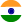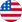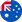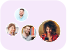24*7 support on WhatsAppChat NowProduct Edition:1st Edition
Author: Richard Haberman
Book Name: Mathematical Models
Subject Name: Maths

# Mathematical Models 1st Edition Solutions

0 out of 5.0
38 reviews21 Students
have requested for homework help from this book

Mathematics is a grand subject in the way it can be applied to various problems in science and engineering. To use mathematics, one needs to understand the physical context. The author uses mathematical techniques along with observations and experiments to give an in-depth look at models for mechanical vibrations, population dynamics, and traffic flow. Equal emphasis is placed on the mathematical formulation of the problem and the interpretation of the results. In the sections on mechanical vibrations and population dynamics, the author emphasizes the nonlinear aspects of ordinary differential equations and develops the concepts of equilibrium solutions and their stability. He introduces phase plane methods for the nonlinear pendulum and for predator-prey and competing species models.

5
19
4
8
3
9
2
8
1
0

0

## Students who viewed this book also checked out